# Using the law of reflection – Ray diagram rules

When we observe an object in a convex mirror there are three ways to try to work out how the light rays from an object focus into the eye of an observer.

1. Use the ray diagram flat/plane mirror rules
2. Use the laws of reflection
3. Use new ray diagram rules and create a virtual image

Here we shall discuss method 2, using the laws of reflection.

In the following diagram we will try to find the light rays from an object which travel to a mirror and then reflect from a mirror and then focus in the eye of an observer. Below shows you a convex mirror, an object and an observer.

Make the observer sit on the principal axis in line with the centre of curvature.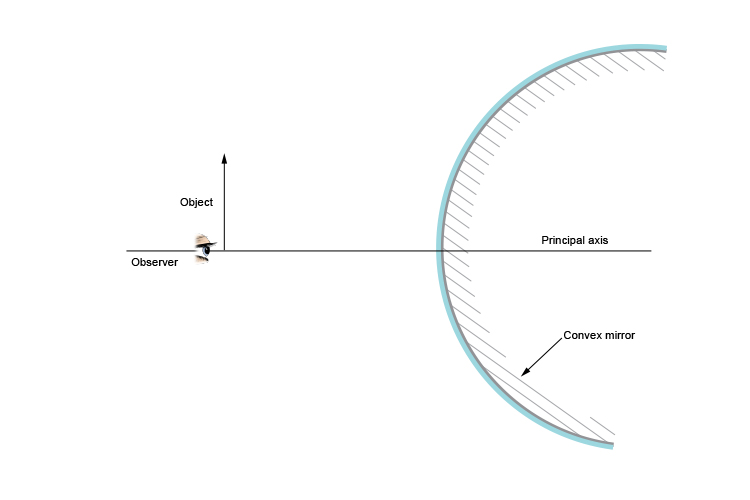Place a protractor with the straight edge against the convex mirror. Use a ruler to extend the protracted lines. Move the protractor around the outside edge of the convex mirror until you have the point of the arrow and the observer with an equal angle between these rays and the 90° mark on the protractor. Always keep the protractor at a tangent (see Mammoth Memory Maths) to the convex mirror.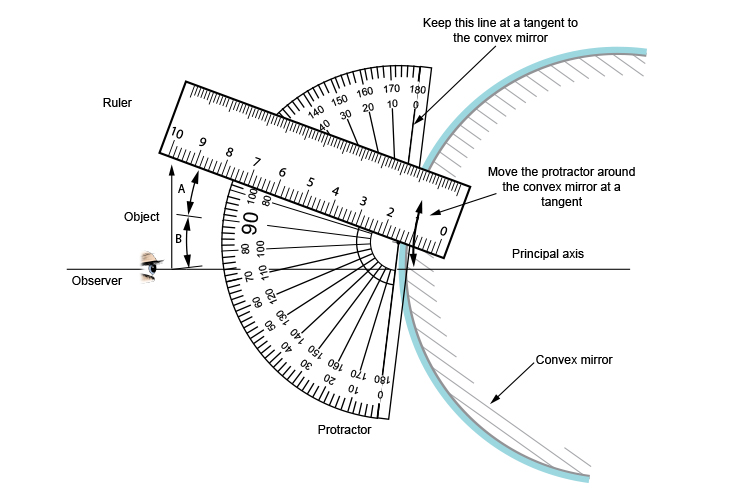Move the protractor until these two angles "A" and "B" are the same.

As you can see (or, hopefully, as you have experimented) aligning the ruler, protractor and mirror and trying to work out an equal angle either side of the 90° on the protractor is difficult and time-consuming.

This concept uses the main law of refraction which states:

The angle between the incident ray and the normal is equal to the angle between the reflected ray and the normal.

When the angle between the incident ray and the normal is equal to the angle between the reflected ray and the normal we can then mark a dot on the outside edge of the protractor where this occurs. You can then draw the incident ray to the mirror and the reflected ray to the observer.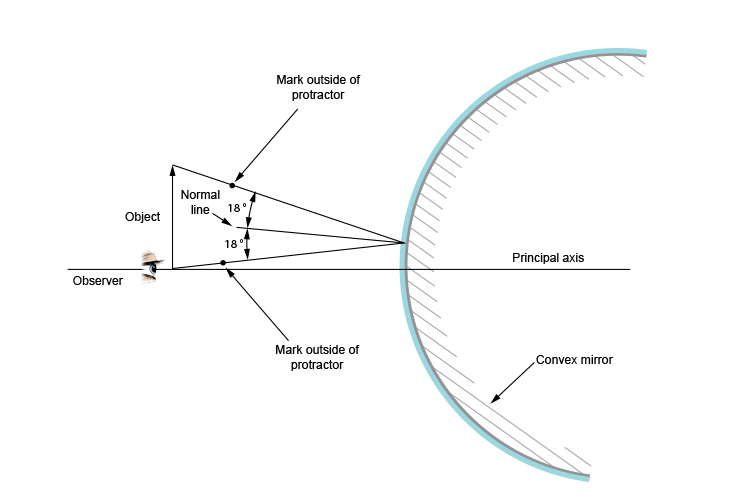Now we have to think about the bottom of the object. The incident ray and the reflected ray will be in the same plane because it is in line with the centre of curvature.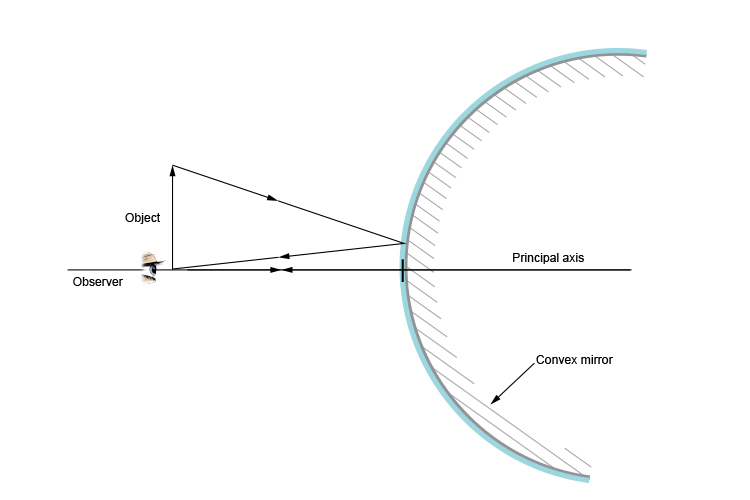Here we have the final ray diagram.

NOTE: This method will work for any object, observer and convex mirror. It will work for any object at any angle and at any distance away from the mirror. All you must do is set the principal axis from the observer to the centre of curvature of the convex mirror.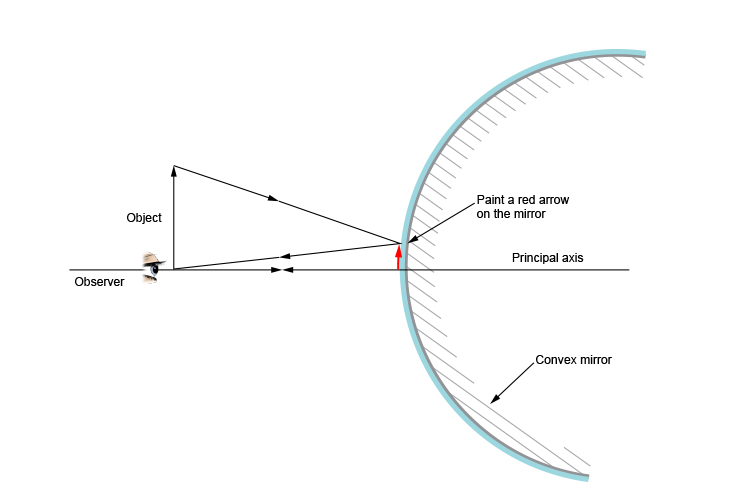If you could ask someone to paint, in red, where the arrow was on the convex mirror it would look like the above diagram.

If you removed the object and left the red arrow, it would look like the original object was still there.

## Conclusion

The above is a very time-consuming method for finding the projected rays from an object.

But why bother when there is a much easier method for finding these lines from convex mirror?

Some very smart scientists set up ray diagram rules for convex mirrors and these will be shown in the next section.

NOTE: The next section's rules are all about creating an imaginary (what people call virtual) image behind the mirror.

The virtual image is created only to find reflected rays from the real object to the observer.

The method shown in the next section is a lot easier to use.